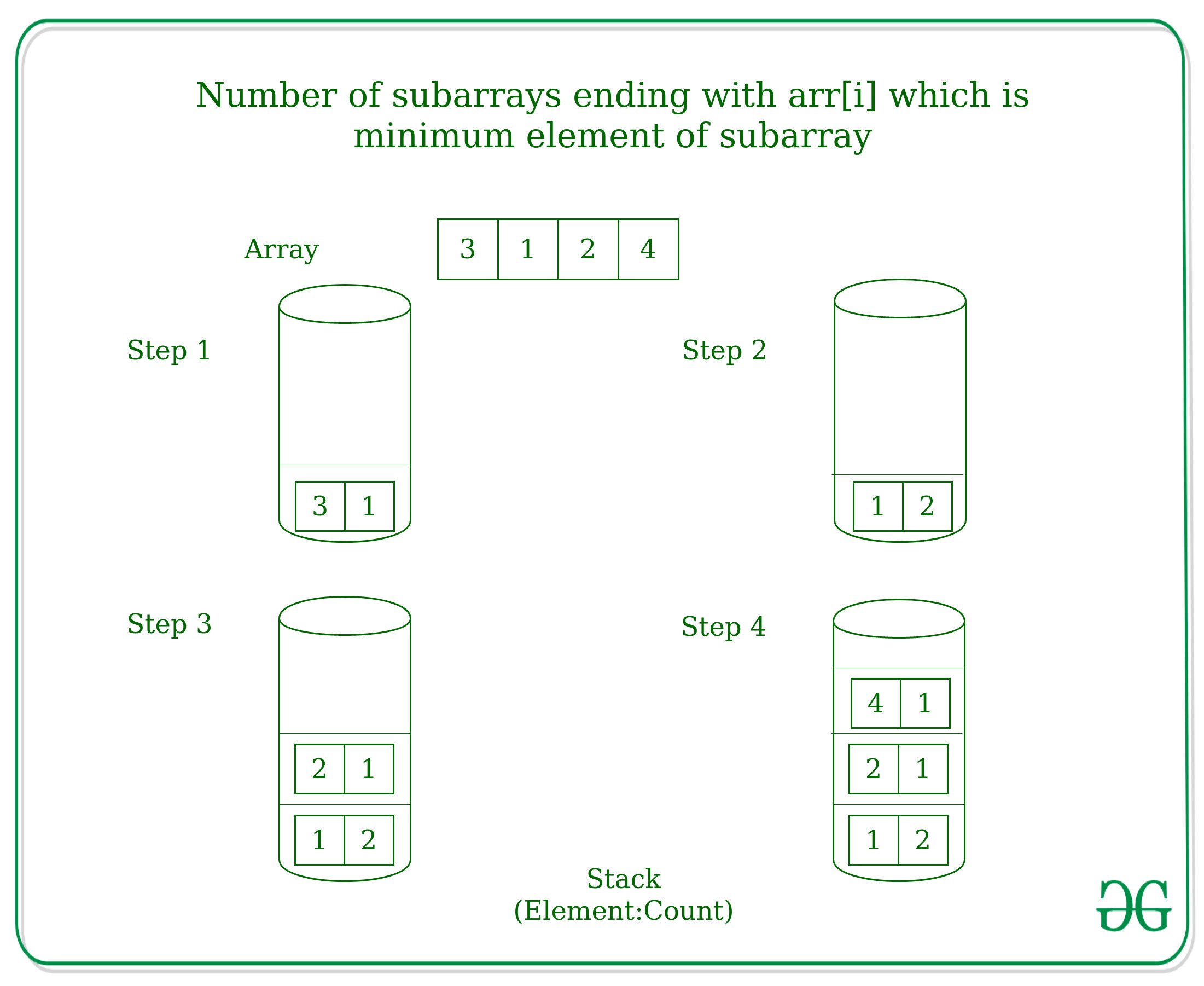Related Articles
Find number of subarrays ending with arr[i] where arr[i] is the minimum element of that subarray
• Last Updated : 03 May, 2021

Given an array, arr[] of size N, the task is to find the number of sub-arrays ending with arr[i] and arr[i] is the minimum element of that sub-array.
Examples:

Input: arr[] = {3, 1, 2, 4}
Output: 1 2 1 1
Explanation:
Subarrays ending with 3 where 3 is the minimum element = {3}
Subarrays ending with 1 where 1 is the minimum element = {3, 1}, {1}
Subarrays ending with 2 where 2 is the minimum element = {2}
Subarrays ending with 4 where 4 is the minimum element = {4}
Input: arr[] = {5, 4, 3, 2, 1}
Output: 1 2 3 4 5

Approach: The idea is to use the approach used to find Next Greater Element by maintaining a stack. Step-wise approach for the problem is:

• Push the first element (arr) of the array with count as 1 in the stack because first element will be the sub-array itself ending with current element arr and minimum of the subarray
• Then for each element arr[i] in the array-
1. Pop the elements from the stack until the top of the stack is greater than the current element and add the count of the popped element in the count of current element.
2. Push current element and the count as a pair in the stack.

For example: For arr[] = {3, 1, 2, 4},Below is the implementation of the above approach:

## C++

 `// C++ implementation to find the number``// of sub-arrays ending with arr[i] which``// is the minimum element of the subarray` `#include ``using` `namespace` `std;` `// Function to find the number``// of sub-arrays ending with arr[i] which``// is the minimum element of the subarray``int` `min_subarray(``int` `a[], ``int` `n)``{``    ``stack > st;``    ` `    ``for` `(``int` `i = 0; i < n; ++i) {``        ` `        ``// There exists a subarray of``        ``// size 1 for each element``        ``int` `count = 1;` `        ``// Remove all greater elements``        ``while` `(!st.empty() &&``               ``st.top().first > a[i]) {``            ` `            ``// Increment the count``            ``count += st.top().second;` `            ``// Remove the element``            ``st.pop();``        ``}` `        ``// Push the current element``        ``// and it's count``        ``st.push({ a[i], count });` `        ``cout << count << ``" "``;``    ``}``}` `// Driver Code``int` `main()``{``    ``int` `a[] = {5, 4, 3, 2, 1};``    ``int` `n = ``sizeof``(a) / ``sizeof``(a);` `    ``min_subarray(a, n);` `    ``return` `0;``}`

## Java

 `// Java implementation to find the number``// of sub-arrays ending with arr[i] which``// is the minimum element of the subarray``import` `java.util.*;``import` `java.lang.*;``import` `java.io.*;` `class` `Main``{``    ``static` `class` `Pair``    ``{``        ``int` `first;``        ``int` `second;``        ``public` `Pair(``int` `x, ``int` `y)``        ``{``            ``this``.first = x;``            ``this``.second = y;``        ``}``    ``}``    ` `// Function to find the number``// of sub-arrays ending with arr[i] which``// is the minimum element of the subarray``static` `void` `min_subarray(``int` `[]a, ``int` `n)``{``    ``Stack st = ``new` `Stack();``    ` `    ``for` `(``int` `i = ``0``; i < n; ++i)``    ``{``        ` `        ``// There exists a subarray of``        ``// size 1 for each element``        ``int` `count = ``1``;` `        ``// Remove all greater elements``        ``while` `(st.empty() == ``false` `&&``            ``st.peek().first > a[i])``        ``{``            ` `            ``// Increment the count``            ``count += st.peek().second;` `            ``// Remove the element``            ``st.pop();``        ``}` `        ``// Push the current element``        ``// and it's count``        ``st.push(``new` `Pair (a[i], count ));` `        ``System.out.print(count + ``" "``);``    ``}``}` `// Driver Code``public` `static` `void` `main(String []args)``{``    ``int` `[]a = {``5``, ``4``, ``3``, ``2``, ``1``};``    ``int` `n = a.length;` `    ``min_subarray(a, n);``}``}` `// This code is contributed by tufan_gupta2000`

## Python3

 `# Python3 implementation to find the number``# of sub-arrays ending with arr[i] which``# is the minimum element of the subarray` `# Function to find the number``# of sub-arrays ending with arr[i] which``# is the minimum element of the subarray``def` `min_subarray(a, n) :` `    ``st ``=` `[];``    ` `    ``for` `i ``in` `range``(n) :``        ` `        ``# There exists a subarray of``        ``# size 1 for each element``        ``count ``=` `1``;` `        ``# Remove all greater elements``        ``while` `len``(st) !``=` `0` `and` `st[``-``1``][``0``] > a[i] :``            ` `            ``# Increment the count``            ``count ``+``=` `st[``-``1``][``1``];` `            ``# Remove the element``            ``st.pop();` `        ``# Push the current element``        ``# and it's count``        ``st.append(( a[i], count ));` `        ``print``(count,end``=` `" "``);` `# Driver Code``if` `__name__ ``=``=` `"__main__"` `:` `    ``a ``=` `[``5``, ``4``, ``3``, ``2``, ``1``];``    ``n ``=` `len``(a);` `    ``min_subarray(a, n);` `# This code is contributed by AnkitRai01`

## C#

 `// C# implementation to find the number``// of sub-arrays ending with arr[i] which``// is the minimum element of the subarray``using` `System;``using` `System.Collections.Generic;` `class` `GFG``{``    ``class` `Pair``    ``{``        ``public` `int` `first;``        ``public` `int` `second;``        ``public` `Pair(``int` `x, ``int` `y)``        ``{``            ``this``.first = x;``            ``this``.second = y;``        ``}``    ``}``    ` `// Function to find the number``// of sub-arrays ending with arr[i] which``// is the minimum element of the subarray``static` `void` `min_subarray(``int` `[]a, ``int` `n)``{``    ``Stack st = ``new` `Stack();``    ` `    ``for` `(``int` `i = 0; i < n; ++i)``    ``{``        ` `        ``// There exists a subarray of``        ``// size 1 for each element``        ``int` `count = 1;` `        ``// Remove all greater elements``        ``while` `(st.Count != 0 &&``            ``st.Peek().first > a[i])``        ``{``            ` `            ``// Increment the count``            ``count += st.Peek().second;` `            ``// Remove the element``            ``st.Pop();``        ``}` `        ``// Push the current element``        ``// and it's count``        ``st.Push(``new` `Pair (a[i], count ));` `        ``Console.Write(count + ``" "``);``    ``}``}` `// Driver Code``public` `static` `void` `Main(String []args)``{``    ``int` `[]a = {5, 4, 3, 2, 1};``    ``int` `n = a.Length;` `    ``min_subarray(a, n);``}``}` `// This code is contributed by Rajput-Ji`
Output:
`1 2 3 4 5`

My Personal Notes arrow_drop_up